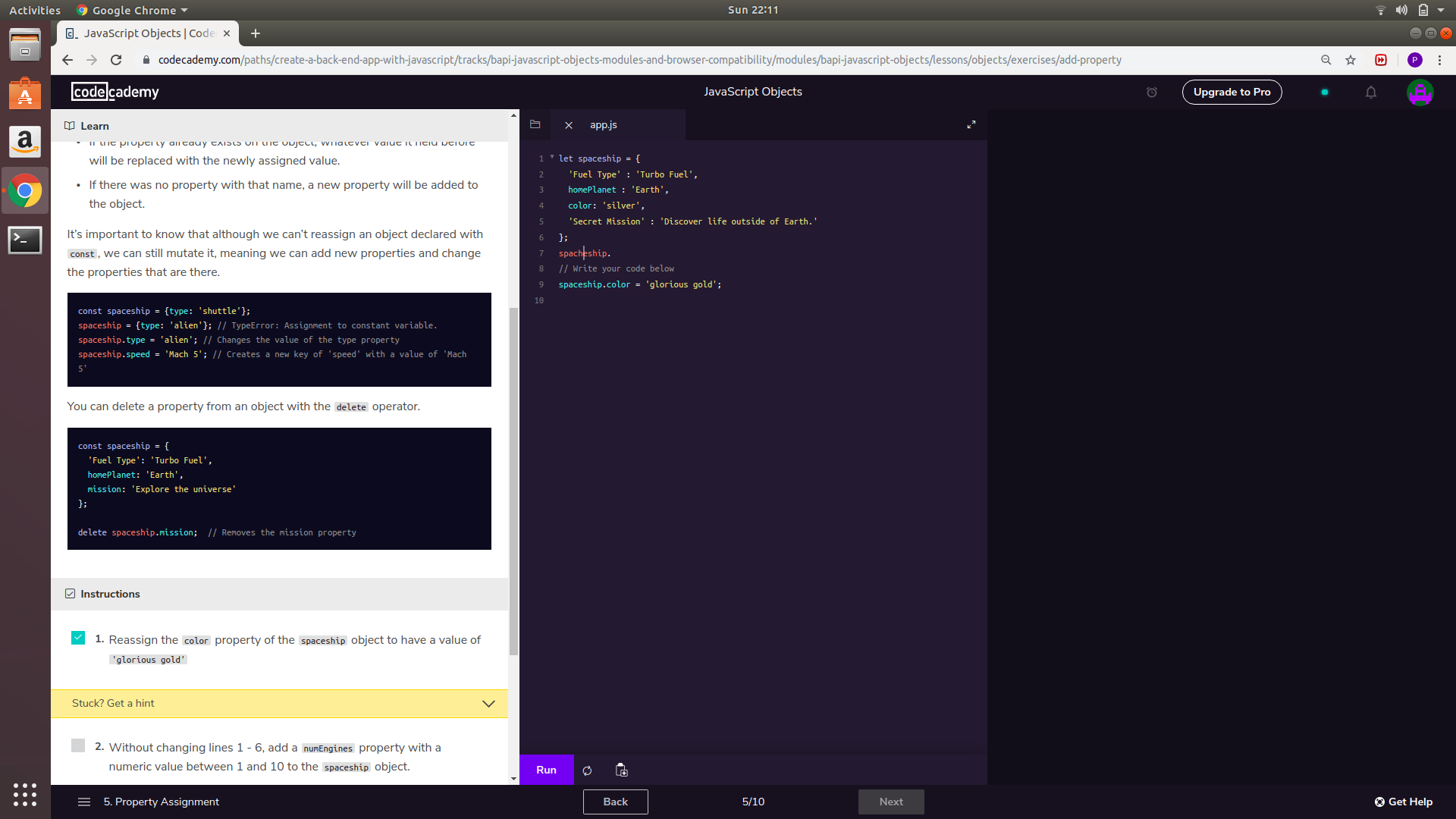# ML | Handle Missing Data with Simple Imputer

SimpleImputer is a scikit-learn class which is helpful in handling the missing data in the predictive model dataset. It replaces the NaN values with a specified placeholder.
It is implemented by the use of the SimpleImputer() method which takes the following arguments :

missing_data : The missing_data placeholder which has to be imputed. By default is NaN
stategy : The data which will replace the NaN values from the dataset. The strategy argument can take the values – ‘mean'(default), ‘median’, ‘most_frequent’ and ‘constant’.
fill_value : The constant value to be given to the NaN data using the constant strategy.

Code: Python code illustrating the use of SimpleImputer class.

 `import` `numpy as np ` ` `  `# Importing the SimpleImputer class ` `from` `sklearn.impute ``import` `SimpleImputer ` ` `  `# Imputer object using the mean strategy and  ` `# missing_data type for imputation ` `imputer ``=` `SimpleImputer(missing_data ``=` `np.nan,  ` `                        ``strategy ``=``'mean'``) ` ` `  `data ``=` `[[``12``, np.nan, ``34``], [``10``, ``32``, np.nan],  ` `        ``[np.nan, ``11``, ``20``]] ` ` `  `print``(``"Original Data : \n"``, data) ` `# Fitting the data to the imputer object ` `imputer ``=` `imputer.fit(data) ` ` `  `# Imputing the data      ` `data ``=` `imputer.transform(data) ` ` `  `print``(``"Imputed Data : \n"``, data) `

Output

```Original Data :
[[12, nan, 34]
[10, 32, nan]
[nan, 11, 20]]

Imputed Data :
[[12, 21.5, 34]
[10, 32, 27]
[11, 11, 20]]
```

Remember : The mean or median is taken along column of the matrix

My Personal Notes arrow_drop_upCheck out this Author's contributed articles.

If you like GeeksforGeeks and would like to contribute, you can also write an article using contribute.geeksforgeeks.org or mail your article to contribute@geeksforgeeks.org. See your article appearing on the GeeksforGeeks main page and help other Geeks.

Please Improve this article if you find anything incorrect by clicking on the "Improve Article" button below.

Article Tags :
Practice Tags :

Be the First to upvote.

Please write to us at contribute@geeksforgeeks.org to report any issue with the above content.